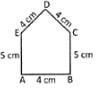# Test : Area - 1

## 10 Questions MCQ Test Mathematics for Class 5: NCERT | Test : Area - 1

Description
Attempt Test : Area - 1 | 10 questions in 10 minutes | Mock test for Class 5 preparation | Free important questions MCQ to study Mathematics for Class 5: NCERT for Class 5 Exam | Download free PDF with solutions
QUESTION: 1

### Length of a rectangle is 2 cm more than that of breadth. If breadth is 16 cm, then find the area of the rectangle.

Solution:

Length = 18cm

Area = l * b

= 18 * 16 = 288cm2

QUESTION: 2

### If perimeter of a square is 52 cm, then find the area of the square.

Solution:

Perimeter of the square = 52cm

Perimeter of the square = side × 4 = 52cm

► side=52/4

► side=13cm

Therefore area of the square = side×side

= 13×13

= 169cm²

QUESTION: 3

### Base of a triangle is 4 cm less than its height. If height is 18 cm, then find the area of the triangle.

Solution:

Given that Base + 4 = Height ... ...(as base is 4 cm less than height)

► And Height = 18 cm

► So Base + 4 = 18 cm

► Base = 18 - 4 = 14 cm

So Area of triangle = ( base × height) / 2 = 126 cm2.

QUESTION: 4

Find the circumference of the circle whose radius is 5.6 cm.

Solution:

Given that the radius r = 5.6 cm

Circumference of a circle = 2 π r = 2 x π x 5.6 = 35.19 cm ≈ 35.2 cm

QUESTION: 5

Find the perimeter of the following figure.Solution:

Perimeter = Sum of all sides

Here the sides are 4 cm , 4 cm , 5 cm , 4 cm and 5 cm

Sum of all sides = 4 + 4 + 5 + 4 + 5 = 22 cm

QUESTION: 6

Perimeter of a rectangle and a square are equal. If perimeter of the rectangle is 72 cm, find the area of the square.

Solution:

Given that Perimeter of rectangle is = 72 cm

► Perimeter of square = 4 x Side of a square

Given that perimeter of a rectangle and a square are equal.

► So 72 cm = 4 x Side of square

► Side of square = 72 / 4 = 18 cm

Now Area of Sqaure = ( side )2

► ( 18 )= 324 cm2

QUESTION: 7

Length, breadth and height of a water tank are 12 m, 15 m, and 18 m respectively. Find the amount of water that the tank can hold.

Solution:

For finding the amount of water, we need to find the volume of the container

Volume of the container = Lenght x Breadth x Height

= 12m x 15m x 18m = 3240m3

QUESTION: 8

Height of a triangle is 3 times than the base. If base of the triangle is 36 cm, find area of the triangle.

Solution:

Given that the base of triangle = 36 cm

► Height = 3 times base = 3 x 36 = 108 cm

► Area of the triangle = (b x h) / 2

► (108 x 36) / 2 = 1944cm2

QUESTION: 9

Radius of a circle is 10.5 cm. Find the area of the circle.

Solution:

So the Area of a circle = π r= π x 10.52 = 346.36 cm2

QUESTION: 10

Area of a square is 10000cm2. Find the perimeter of the square.

Solution:

Area of square = 10000cm2 = side x side

= Side = 100 cm

Perimeter of the Square = 4 x side = 4 x 100 = 400 cmUse Code STAYHOME200 and get INR 200 additional OFF Use Coupon Code